# Sample Data: Employee Attitude Survey

Employee attitude data for 30 departments in a large financial organization

Originator: S. Chatterjee and B. Price

Each observation is an aggregate of questionnaire responses from approximately 35 randomly selected employees in a department. The numbers give the percentage of favorable responses to seven questions.

## Examples

### Basic Examples

Retrieve the ResourceObject:

 In:=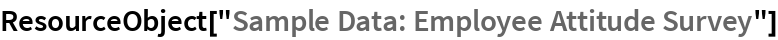Out=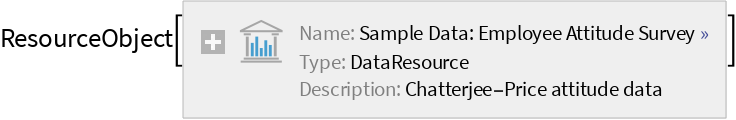View the data:

 In:=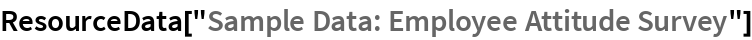Out=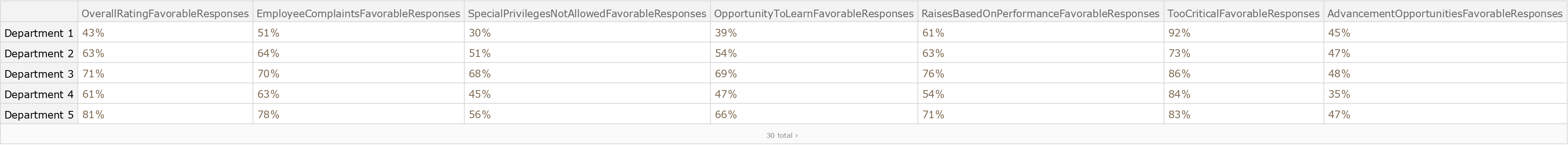### Analysis

Calculate the median positive response rate for each survey question:

 In:=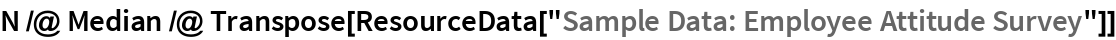Out=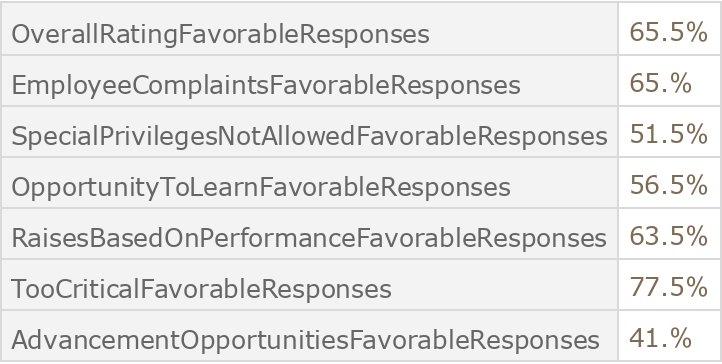Wolfram Research, "Sample Data: Employee Attitude Survey" from the Wolfram Data Repository (2016) https://doi.org/10.24097/wolfram.10151.data